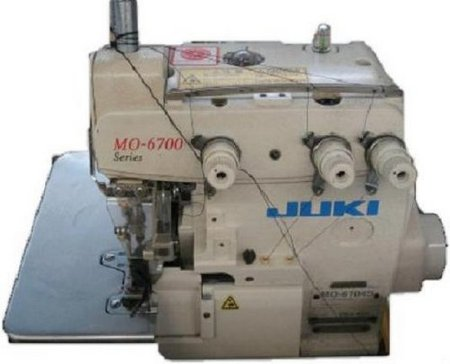# Textile Calculation

Problems and solutions of textile

## Consumption Formula for 3-Thread Overlock Sewing Machine

Consumption Formula for 3-Thread Overlock Sewing Machine
Noor Ahmed Raaz
B.Sc. in Apparel Manufacturing
Asst. Merchandiser at A.M.C.S Textile Ltd
Email: raju.uttara72@yahoo.com

3-Thread Overlock Sewing Machine:

This machine is manufactured by Juki, a world leader in sewing quality and technology, this machine is intended solely for overlock stitching of light to medium weight fabrics. It is designed to perform at high speed with excellent reliability and consistancy.3-Thread Overlock Sewing Machine
Consumption formula for 3 threads Over locks sewing:C = (3N + 4W + 4T) × S (Here, W = Seam width.)

= 3NS + 4WS + 4TS = 3 + 4S (W + T); Because NS =1.

Problem-1:

(a) If there are 10 stitches per inch thickness are 0.050″ and seam width 0.25″, then what will be threading Consumption per inch?

Solution: C = 3 + 4S (W + T) = 3 + 4 ×10 × (0.25″ + 0.050″) = 15″ (Ans.)

Problem-2:

(b) If there are 20 yds. Of Over lock sewing in a garment then how many meters of threads are needed for Over lock sewing?

Solution:

1yds. = 36 inch

Then, 20 yds. = 36 × 20 = 720 inch.

1″ needed = 15 thread

Then, 720″ needed = 15 × 720 thread

= 10800 thread.

Need thread consumption = 10800/39.37 = 274.32 meter. (Ans.)